# 氘氚气体与CO2在常温下的辐照性能研究Researches on Irradiation Properties of Deuterium-Tritium Mixed Gases with CO2 in Room Temperature

• 全文下载: PDF(2155KB)    PP.55-60   DOI: 10.12677/NST.2018.63007
• 下载量: 478  浏览量: 878   科研立项经费支持

Pressure monitoring, quadrupole mass spectrometer, Raman spectrum, color spectrum, and the-oretical arithmetic were carried out on the reaction deuterium-tritium mixed gases and CO2. The results declare that the pressure variation of the reaction system is not patency, and there is no CO engender in reaction initial stage (<2 min). With the reaction proceeding (>10 min), the pressure variation of the reaction system becomes downtrend and CO starts producing, and the CO output increases with the raise of the temperature and tritium content. The theoretical arithmetic results show that the possibility for CO2 and T2 direct reaction is low, but CO2 can react with T2 under the effect of T+ generated by β irradiation, and CO is the main product of those reactions. Ionic molecule reactions are the main reactions for CO2 and T2 system, and the experimental and theoretical results of reaction rate make a good accordance.

1. 引言

2. 实验过程

2.1. 实验工装的体积标定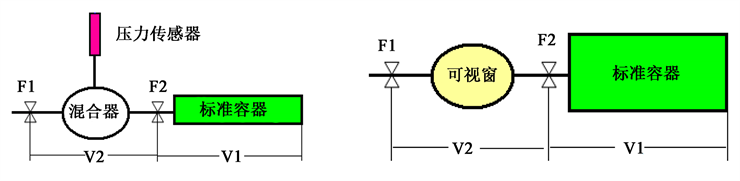Figure 1. Individualistic experimental overalls (Left: pressure monitoring, right: composition analysis)

2.2. 实验工装的热除气

3. 结果与讨论

3.1. 氘与CO2共存时的压力变化

3.2. 氘氚气体与CO2的共存时的压力变化

3.3. 氘氚气体与CO2的共存时的气相成分变化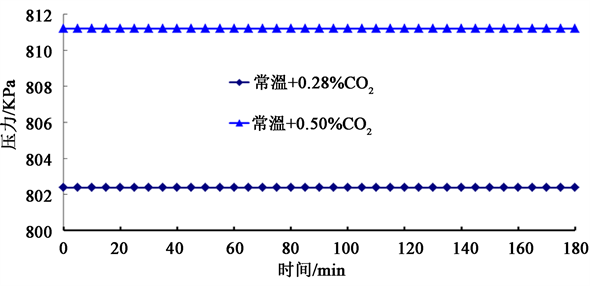Figure 2. Pressure variation of D2 and CO2 coexist systemFigure 3. Pressure vary with time of reaction system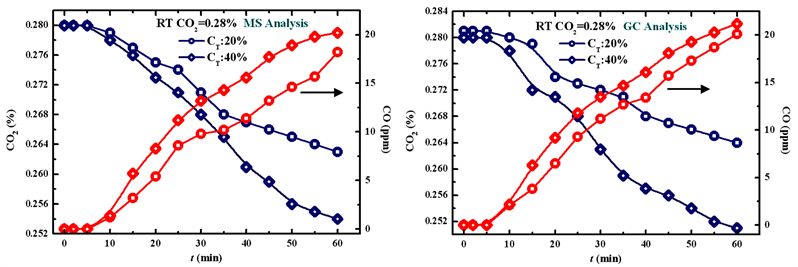Figure 4. Concentration variation of different proportion deuterium and tritium gases coexist with 0.28% CO2

3.4. 氘氚气体与CO2的共存时的理论计算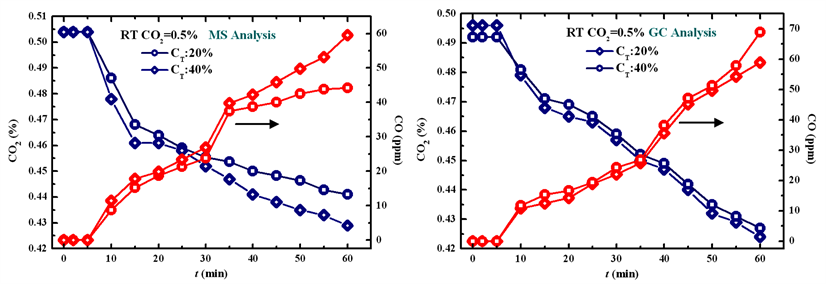Figure 5. Concentration variation of different proportion deuterium and tritium gases coexist with 0.5% CO2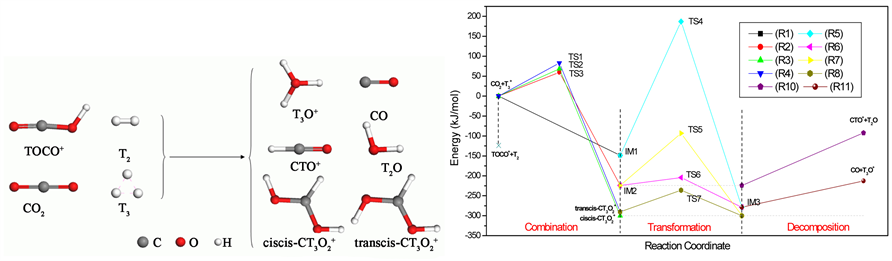Figure 6. Possible structures of reactants and products and energy path for CO2+T3+ system

${\text{T}}_{\text{2}}\stackrel{\beta \text{\hspace{0.17em}}\text{decly}}{\to }{\left[{}^{\text{3}}\text{H}\text{eT}\right]}^{+}+{e}^{-}\to {\text{T}}^{+}+{}^{\text{3}}\text{H}\text{e}+{e}^{-}$ (1)

${\text{T}}^{+}+{\text{T}}_{2}\to {\text{T}}_{3}^{+}\text{ }\Delta H=-411.5\text{\hspace{0.17em}}\text{kJ}/\text{mol}$ (2)

${\text{T}}^{+}+{\text{CO}}_{2}\to {\text{TOCO}}^{+}\text{ }\Delta H=-535.0\text{\hspace{0.17em}}\text{kJ}/\text{mol}$ (3)

${\text{CO}}_{2}+{\text{T}}_{3}^{+}\to \text{TS}1\to \text{IM}2\to \text{TS}5\to \text{IM}3\to \text{CO}+{\text{T}}_{\text{3}}{\text{O}}^{+}$ 反应速率：8.76 × 10−7 s−1

4. 结论

1) 氘氚气体与CO2短期共存时，反应初期(2 min内)，体系总压、氚和CO2的含量均无明显变化，在10 min后，体系总压、氚和CO2含量均逐渐降低并伴随有CO的生成，其生成量随体系氚含量和CO2的增加而呈现上升的趋势。

2) 氘氚气体中含有一定比例的 ${\text{T}}_{3}^{+}$ 分子，且随着氚贮存时间增长，体系中 ${\text{T}}_{3}^{+}$ 分子浓度也将增加，CO2${\text{T}}_{3}^{+}$ 分子的反应具有最低的能垒，是最有可能发生的反应。T+在反应过程中起类似催化的作用，从最初的与T2结合形成 ${\text{T}}_{3}^{+}$ ，到最后于T2O结合形成水合氢离子T3O+，T+在反应过程中并没有消耗，产物T3O+在一定条件下可继续参与反应。

3) 根据Arrhenius方程可求得标准状况下CO2${\text{T}}_{3}^{+}$ 分子反应速率约为8.76 × 10−7 s−1，其值与实验结果的符合性较好，且远高于氚的衰变速率1.77 × 10−9 s−1。即氚衰变产生的T+是引起氚与二氧化碳反应的主要原因，而非辐照裂解。

  Penzhorn, R.D., Berndt, U., Caldwell-Nichols, C., et al. (2000) Radiochemistry of an Equimolar Deuterium-Tritium Mixture or of T2 with CO. Fusion Engineering & Design, s49-50, 927-937.  O’Hira, S., Nakamura, H. and Okuno, K. (1995) Beta Decay Induced Reaction Studies of Tritium by Laser Raman Spectroscopy. Fusion Technology, 28, 1239-1243. https://doi.org/10.13182/FST95-A30579  Douglas, D.L. (1995) Tritium-Carbon Monoxide Reaction. The Journal of Chemical Physics, 23, 1558-1562.  Federici, G., Anderl, R.A., Andrew, P., et al. (2001) In-Vessel Tritium Retention and Removal in JET. Journal of Nuclear Materials, 266, 14-29.  O’Hira, S., Isobe, K., Suzuki, T., et al. (2000) Beta Induced Reaction Study on T2-CO System. Fusion Engineering & Design, s49-50, 905-914.  Shu, W.M., O’Hira, S., Suzuki, T., et al. (2004) Radiochemical Reactions between Tritium Oxides and Carbon Monoxide. Fusion Engineering & Design, 70, 123-129. https://doi.org/10.1016/j.fusengdes.2003.09.001  Sherman, R.H., Taylor, D.J., Honnell, K.G., et al. (1997) Radiochemical Reactions between Tritium and Humid Air. IEEE/NPSS Symposium on Fusion Engineering, 1, 313-319.  Wang, J., Li, J., Ma, J.Y., et al. (2014) Full-Dimensional Characterization of Photoelectron Spectra of HOCO- and DOCO- and Tunnelling Facilitated Decay of HOCO Prepared by Anion Photodetachment. The Journal of Chemical Physics, 140, 184314. https://doi.org/10.1063/1.4874975  Zhang, S.S., Medvedev, D.M., Goldfield, E.M., et al. (2006) Quantum Dynamics Study of the Dissociative Photodetachment of HOCO-. The Journal of Chemical Physics, 125, 4312. https://doi.org/10.1063/1.2360945  Li, R. and Ao, K.F. (2002) A Study on the Reaction of Reaction of OH Radical with CO by Time-Resolved FTIR Spectroscopy. Acta Physico-Chimica Sinica, 18, 486-489.